# Precalculus : Graph a Linear Function

## Example Questions

### Example Question #1 : Graph A Linear Function

Which of the following could be the function modeled by this graph?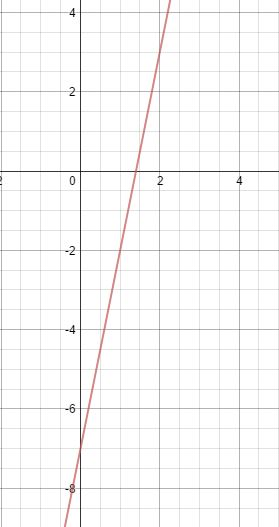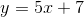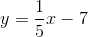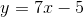Explanation:

Which of the following could be the function modeled by this graph?We can begin here by trying to identify a couple points  on the graph

We can see that it crosses the y-axis at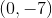Therefore, not only do we have a point, we have the y-intercept. This tells us that the equation of the line needs to have ain it somewhere. Eliminate any option that do not have this feature.

Next, find the slope by counting up and over from the y-intercept to the next clear point.

It seems like the line goes up 5 and right 1 to the point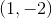This means we have a slope of 5, which means our equation must look like this: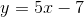### Example Question #2 : Graph A Linear Function

Find the slope of the linear function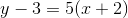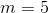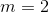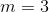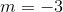Explanation:

For the linear function in point-slope form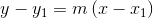The slope is equal to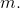For this problemwe get### Example Question #3 : Graph A Linear Function

Find the slope of the linear function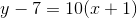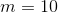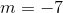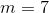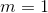Explanation:

For the linear function in point-slope formThe slope is equal toFor this problemwe get### Example Question #51 : Graphing Functions

What is the y-intercept of the line below?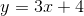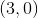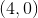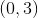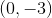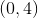Explanation:

By definition, the y-intercept is the point on the line that crosses the y-axis. This can be found by substitutinginto the equation. When we do this with our equation,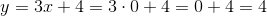Alternatively, you can rememberform, a general form for a line in whichis the slope andis the y-intercept.

### Example Question #1 : Linear Functions

What is the slope of the line below?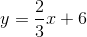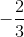Explanation:

Recall slope-intercept form, or. In this form,is the slope andis the y-intercept. Given our equation above, the slope must be the coefficient of the x, which is### Example Question #6 : Graph A Linear Function

What is the x-intercept of the equation below?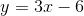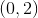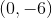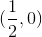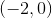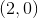Explanation:

The x-intercept of an equation is the point at which the line crosses the x-axis. Thus, we can find the x-intercept by plugging in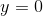. When we do this with our equation: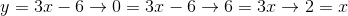Thus, our x-intercept is the point### All Precalculus Resources